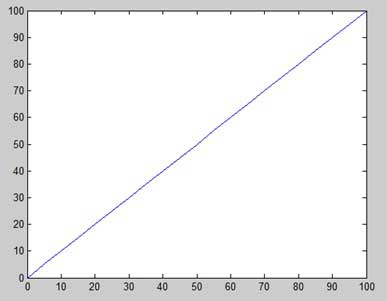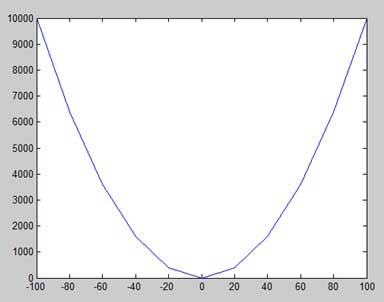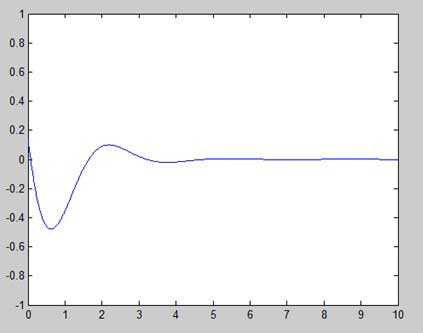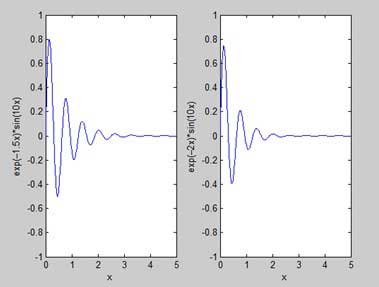# MATLAB - Plotting

To plot the graph of a function, you need to take the following steps −

• Define x, by specifying the range of values for the variable x, for which the function is to be plotted

• Define the function, y = f(x)

• Call the plot command, as plot(x, y)

Following example would demonstrate the concept. Let us plot the simple function y = x for the range of values for x from 0 to 100, with an increment of 5.

Create a script file and type the following code −

```x = [0:5:100];
y = x;
plot(x, y)
```

When you run the file, MATLAB displays the following plot −Let us take one more example to plot the function y = x2. In this example, we will draw two graphs with the same function, but in second time, we will reduce the value of increment. Please note that as we decrease the increment, the graph becomes smoother.

Create a script file and type the following code −

```x = [1 2 3 4 5 6 7 8 9 10];
x = [-100:20:100];
y = x.^2;
plot(x, y)
```

When you run the file, MATLAB displays the following plot −Change the code file a little, reduce the increment to 5 −

```x = [-100:5:100];
y = x.^2;
plot(x, y)
```

MATLAB draws a smoother graph −## Adding Title, Labels, Grid Lines and Scaling on the Graph

MATLAB allows you to add title, labels along the x-axis and y-axis, grid lines and also to adjust the axes to spruce up the graph.

• The xlabel and ylabel commands generate labels along x-axis and y-axis.

• The title command allows you to put a title on the graph.

• The grid on command allows you to put the grid lines on the graph.

• The axis equal command allows generating the plot with the same scale factors and the spaces on both axes.

• The axis square command generates a square plot.

### Example

Create a script file and type the following code −

```x = [0:0.01:10];
y = sin(x);
plot(x, y), xlabel('x'), ylabel('Sin(x)'), title('Sin(x) Graph'),
grid on, axis equal
```

MATLAB generates the following graph −## Drawing Multiple Functions on the Same Graph

You can draw multiple graphs on the same plot. The following example demonstrates the concept −

### Example

Create a script file and type the following code −

```x = [0 : 0.01: 10];
y = sin(x);
g = cos(x);
plot(x, y, x, g, '.-'), legend('Sin(x)', 'Cos(x)')
```

MATLAB generates the following graph −## Setting Colors on Graph

MATLAB provides eight basic color options for drawing graphs. The following table shows the colors and their codes −

Code Color
w White
k Black
b Blue
r Red
c Cyan
g Green
m Magenta
y Yellow

### Example

Let us draw the graph of two polynomials

• f(x) = 3x4 + 2x3+ 7x2 + 2x + 9 and

• g(x) = 5x3 + 9x + 2

Create a script file and type the following code −

```x = [-10 : 0.01: 10];
y = 3*x.^4 + 2 * x.^3 + 7 * x.^2 + 2 * x + 9;
g = 5 * x.^3 + 9 * x + 2;
plot(x, y, 'r', x, g, 'g')
```

When you run the file, MATLAB generates the following graph −## Setting Axis Scales

The axis command allows you to set the axis scales. You can provide minimum and maximum values for x and y axes using the axis command in the following way −

```axis ( [xmin xmax ymin ymax] )
```

The following example shows this −

### Example

Create a script file and type the following code −

```x = [0 : 0.01: 10];
y = exp(-x).* sin(2*x + 3);
plot(x, y), axis([0 10 -1 1])
```

When you run the file, MATLAB generates the following graph −## Generating Sub-Plots

When you create an array of plots in the same figure, each of these plots is called a subplot. The subplot command is used for creating subplots.

Syntax for the command is −

```subplot(m, n, p)
```

where, m and n are the number of rows and columns of the plot array and p specifies where to put a particular plot.

Each plot created with the subplot command can have its own characteristics. Following example demonstrates the concept −

### Example

Let us generate two plots −

y = e−1.5xsin(10x)

y = e−2xsin(10x)

Create a script file and type the following code −

```x = [0:0.01:5];
y = exp(-1.5*x).*sin(10*x);
subplot(1,2,1)
plot(x,y), xlabel('x'),ylabel('exp(–1.5x)*sin(10x)'),axis([0 5 -1 1])
y = exp(-2*x).*sin(10*x);
subplot(1,2,2)
plot(x,y),xlabel('x'),ylabel('exp(–2x)*sin(10x)'),axis([0 5 -1 1])
```

When you run the file, MATLAB generates the following graph −